# HLOOKUP vs VLOOKUP: A Beginner's GuideLydia Uys

## What are HLOOKUP and VLOOKUP functions?

Let’s explore HLOOKUP vs VLOOKUP in Excel, and find out exactly how and when to use each.

The HLOOKUP and VLOOKUP functions are grouped in the lookup category of functions. The function looks for a certain value in the first row (HLOOKUP - (h)orizontal) or column (VLOOKUP - (v)ertical) of a table and returns any value from the same column (HLOOKUP) or row (VLOOKUP).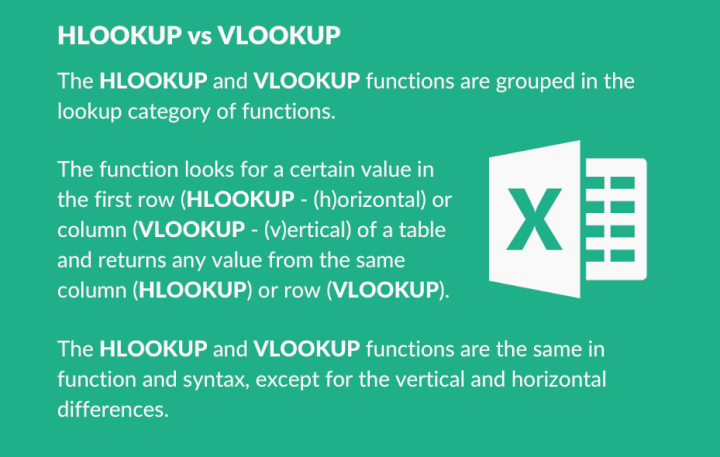In the example below, we are using the Member ID field in the Workshop Registrations dataset to find the same Member ID in the Members dataset, to return the First Name linked to the ID.

A VLOOKUP will be used if the Member IDs are in a column.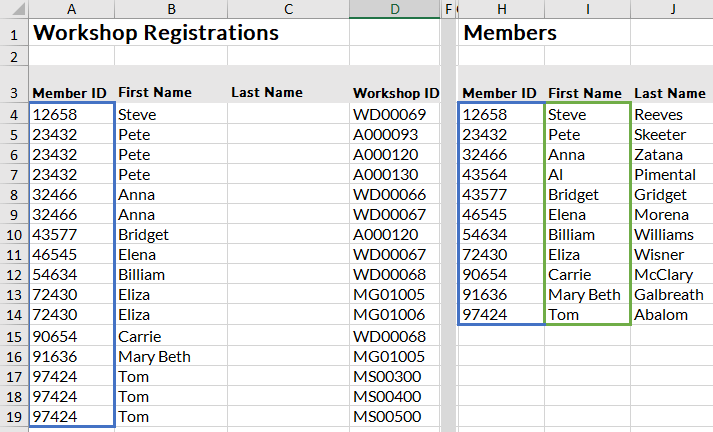The HLOOKUP will be used if the Member IDs are listed in a row.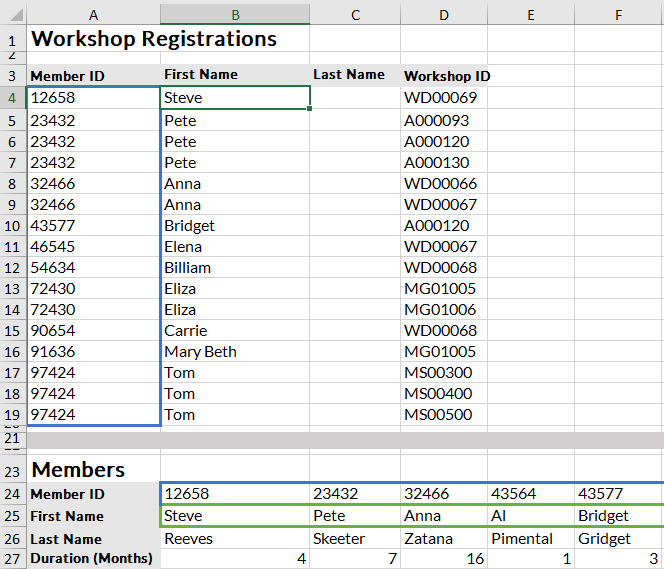Lists are usually in columns, hence VLOOKUP is used more often in practice than HLOOKUP.

Quick Tip: If you have access to Microsoft 365, keep an eye out for the newer and improved XLOOKUP function. XLOOKUP is not available to all versions of Excel yet, but if you think H/VLOOKUP is handy, you'll want to learn about XLOOKUP. Check out our XLOOKUP resource for more information and examples of this new function.

## How does each function work?

=VLOOKUP(lookup_value, table_array, col_index_num, [range_lookup])

=HLOOKUP(lookup_value, table_array, col_index_num, [range_lookup])

Lookup_ value:

• What do you want to find?

Table_array:

• Where do you want to find it?
• The column or row potentially containing the lookup value must be in the left column of this range (VLOOKUP) or the top row (HLOOKUP).
• The column containing the returned value should also be included in the range.

Col_index_num:

• The column in the table_array to be returned.

Range_lookup:

• [Brackets] indicating optional argument.
• False or exact match will search for a value exactly the same as the lookup.
• True or approximate match needs the search column to be sorted in ascending order (small to large) and will return the next smaller value than the one required if that value is not found.

Use this free Excel file to practice along with the tutorial.

## When to use HLOOKUP vs VLOOKUP

### Example 1: HLOOKUP

In the example below, member IDs are spread across rows with details down the column. You will have to use a lookup that searches horizontally - HLOOKUP.

To find the first name of the member for the workshop registration, you would add an HLOOKUP to the column B cell to find the member’s first name from the member’s information below.

In Excel, the HLOOKUP takes the member ID (marked yellow) from A4 and compares it to the first data in the top row of the table array (blue area).

When it finds the member ID in B24, it can now give you any value listed below B24 as long as it is in the defined blue area (B24:L27). The search is not case sensitive, but it is character sensitive.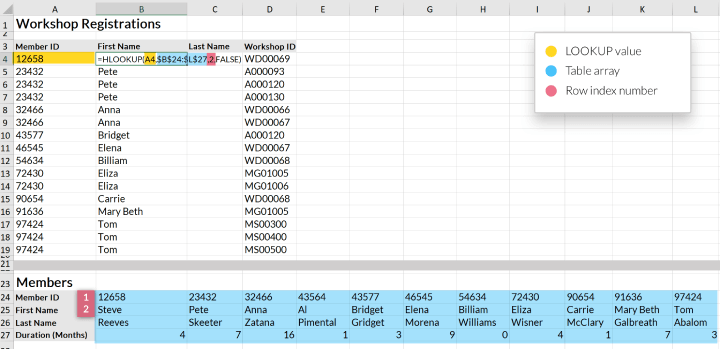### Example 2: VLOOKUP

In the second example, the member IDs are listed in column H (H4:H14) and the relevant information for each member is listed in the row next to this. If the data you want to search in is in a column, a VLOOKUP is needed because you want to search vertically.

The member ID is in A4 (yellow) and the table array is defined in blue (H4:K14). Excel looks for 12658 in the first column of the table array (blue) and finds Steve in I4.

Now Excel can return any value in row 4 as long as it is in the blue area range. The blue area columns are numbered (see the red letters at the top) and this is the number required for the returned value.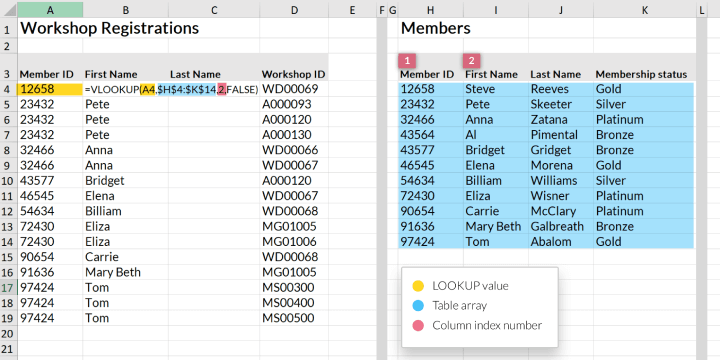### More examples of how it works

#### Exact matches:

The function will look for something exactly like the lookup. If it cannot find something exactly alike, a #N/A will be returned. This will cause no returns if the one value has additional space characters in the cell, or if one value is a number and the search column contains numbers stored as text.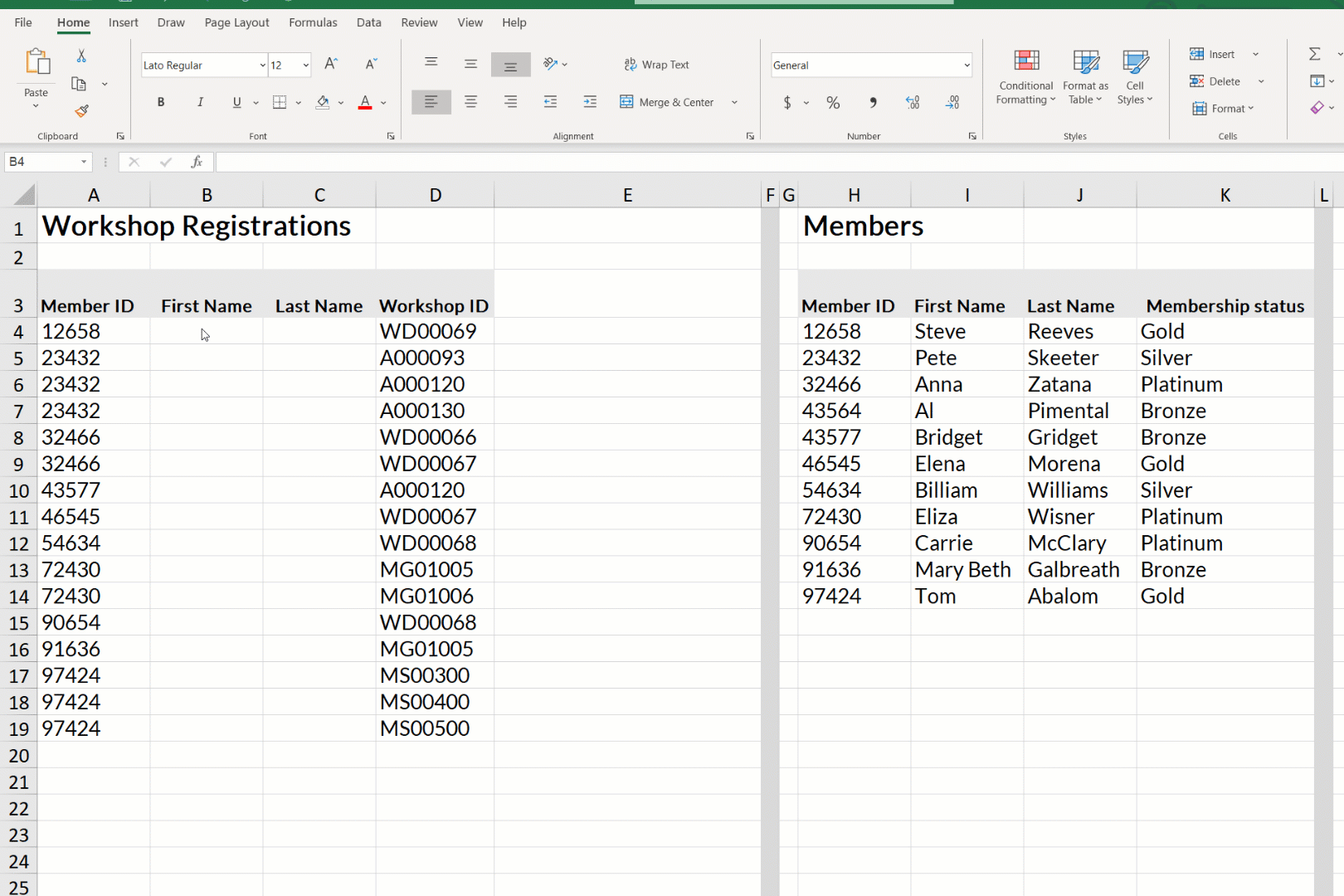To solve this problem, wildcard characters may be employed. These qualify as wildcard characters:

• ?
• ~
• *

The ? would replace one character and the * takes the place of 0 or multiple. Wildcards work with exact matches only.

#### Approximate matches:

This function will return either the exact match or the value just smaller than the one it is looking for. Data in the search column has to be sorted in ascending order for the approximate to return values you require. Including headings in your table may also (in very few instances) give you incorrect returns, so exclude them just to be sure.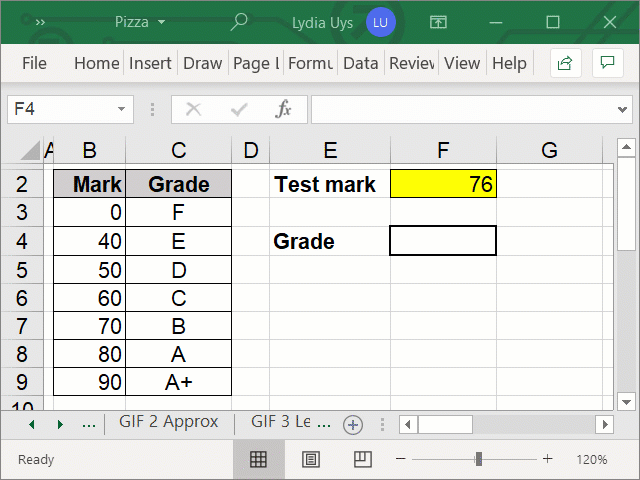#### Limitation of search row or column position:

The limitation of the returned value row (HLOOKUP) or the returned value column (VLOOKUP) position in relation to the lookup value (either below or to the right) poses a problem where the returned value is above or to the left of the lookup values.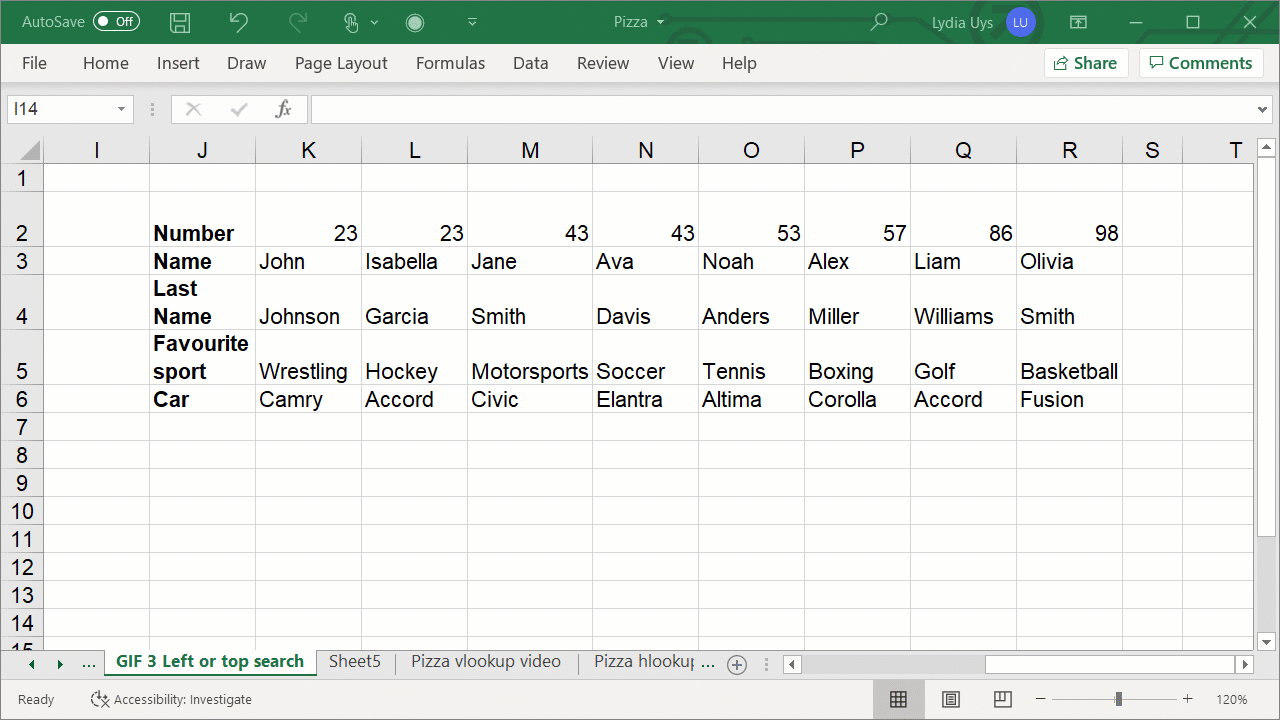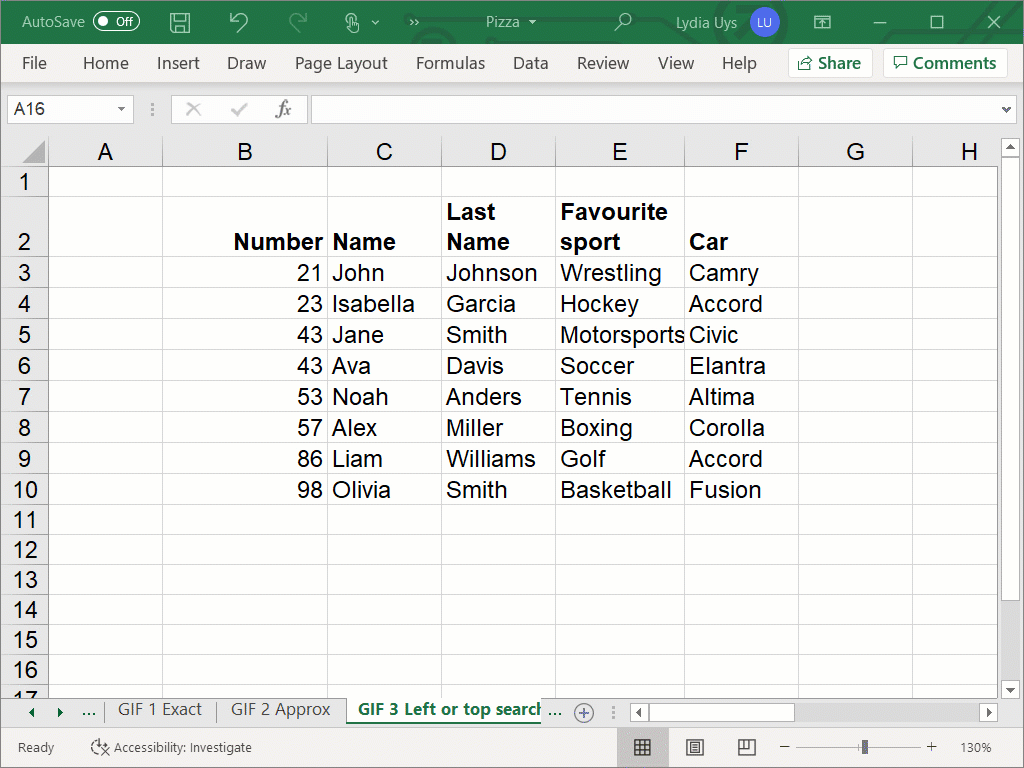This can be overcome by combining a CHOOSE function with the HLOOKUP or VLOOKUP, or by using the INDEX and MATCH function

The CHOOSE function changes the columns around so that the H/VLOOKUP thinks it is searching left and returning columns to the right without actually changing the data.

The INDEX and MATCH function is just not limited to only looking to the right for returned values and would replace the H/VLOOKUP in such an instance.

#### Linear search:

These lookups search from left to right (HLOOKUP) or from top to bottom (VLOOKUP). This may slow your spreadsheet down a little  - the bigger the sheet, the more speed may be an issue.

Recent Microsoft updates focused on the speed issue and it may not even be an issue still or remain one for long.

#### Unique values

Because of the linear search method for these VLOOKUPs, should the search field have duplicate fields, Excel will return only the first value found and will ignore all others. These functions will work best in search fields that contain unique values.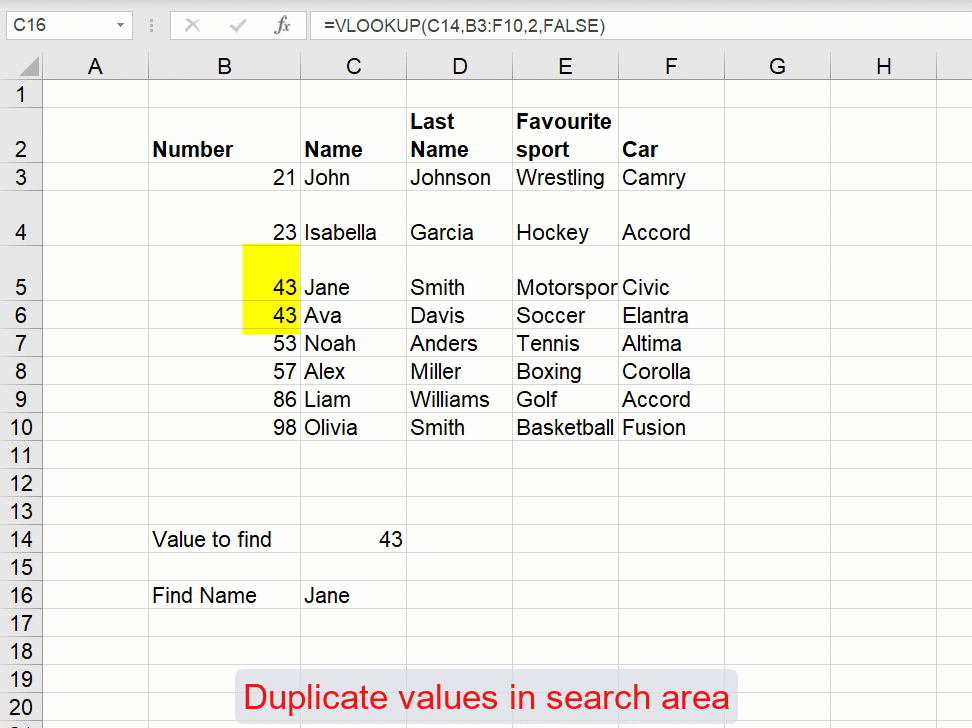## Error messages

#N/A - the value searched for cannot be found - only in exact matches.

In the example below, this would display if you looked for a number (25) that does not exist in the list.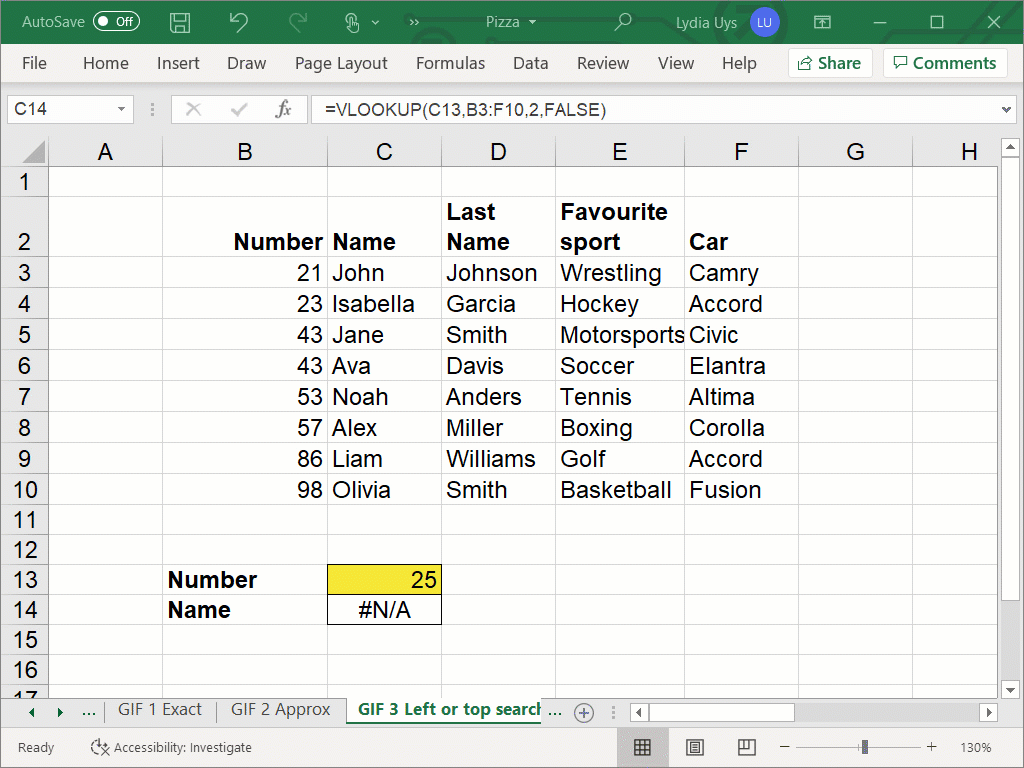If you want a customized message here, you can add an IFERROR to the VLOOKUP and use the return value argument in IFERROR to add a message like “The value is not found.”

#Ref - the returned field required is outside of the defined area.## Conclusion

The HLOOKUP and VLOOKUP functions are the same in function and syntax, except for the vertical and horizontal differences. It is globally accepted that VLOOKUP is one of the handiest functions available in Excel.

If you are interested in learning more about VLOOKUPs and other essential Excel skills, try our Excel - Basic and Advanced course now. Or you can start with some Excel basics by trying the free Excel in an Hour course.

Free Excel crash course

Learn Excel essentials fast with this FREE course. Get your certificate today!Lydia Uys

Lydia is a Chartered Accountant and has experience in financial management, lecturing, and consulting. She currently creates content for GoSkills and also loves spending time with family and friends.

1 comment

•Tavia

Great functions!